## General Question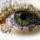# How do I find the amount of possible equations for a certain number?

Asked by Steve_A (5125) May 18th, 2010

Like if I pulled a certain number 256 , or 4,988 etc.

Is there a way to find all possible to ways to get that number? Formula or trick for it?

Thanks.

Observing members: 0Composing members: 0## 14 Answers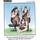Infinity is all the combinations

talljasperman (21866)“Great Answer” (2) Flag as…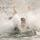There are an infinite number of ways to “get” that number, assuming you’re working with all acceptable mathematical operations (addition, subtraction, multiplication, division, exponents, etc). For example, 255+1, 255+2–1, 255+3–2, etc etc etc.

If you only want to find the factors that you can multiply together to get that number, you might want to look at prime factorization.

hannahsugs (3238)“Great Answer” (5) Flag as…That is what I first thought.

But even if I did just the addition possibilities would it still be infinity?

Steve_A (5125)“Great Answer” (1) Flag as…Yes, it would still be infinity. There are some finite ways that i I can think of, but you have to limit what operations are allowed. For instance, if you limited yourself to multiplication of positive integers, or addition of positive integers, without allowing for other operations in those expressions, there would only be a finite way to do that.

hannahsugs (3238)“Great Answer” (4) Flag as…Dumb question(mind you I suck at math) why would adding more than one operation automatically make it from finite to infinity?

Steve_A (5125)“Great Answer” (1) Flag as…For instance, if you can only add positive integers, you must begin with two numbers that are smaller than the “goal” number. There are a finite number of positive integers smaller than your goal number, so there’s only a finite number of ways to do that. Even if you can add as many positive integers as you want to get to your goal, there’s still only a finite number of ways to do this, starting with numbers that are all smaller than your “goal”. At the most, you’re adding 1+1+1+1+1….. until you get to your goal.

By adding another operation, I mean adding something like subtraction, where previously you only had addition.

If you can add and subtract, you can add ANY two numbers that take your fancy, no matter how big they are, and then subtract whatever third number is necessary to achieve your “goal.” Since there’s an infinite number of pairs of numbers that you can start with, this option would give you an infinite number ways to get your answer.

hannahsugs (3238)“Great Answer” (2) Flag as…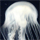A disclaimer: I’m a mathematician, so I hope I’m not confusing the matter by speaking too generally. If I do, please tell me so I can clarify. I don’t bite!

I think part of the problem here is in the way you phrased the question.

Some terminology:
A mathematical expression is a sequence of numbers (or variables, but we won’t go there) connected by operations, something like ”(2+4–8)*7”.

An equation is two expressions with an equals sign in between them, like “3+7=10”.

What it sounds like you’re asking is “Once I fix a given number, how many expressions can I write down that are equal to that fixed number?” If that’s not what you’re asking, please tell me, as I have misunderstood you.

The answer to this question, as @hannahsugs has explained, is that there are infinitely many such expressions. But since that’s not a particularly interesting answer, a mathematician’s instinct is to modify the question slightly and see if you get a more interesting result. If your goal is to impose restrictions on the original problem in order to get a finite answer, there are several ways to go about it.

First, you have to determine how you’re going to count these expressions. While this may seem obvious (“I learned to count in kindergarten, thank you very much”), it actually presents some real difficulties. For example, if you count “3+4”, “3+4+0”, “3+4+0+0”, etcetera, as different expressions, then there is no hope of getting a finite number of possibilities. (Do you see why?) The same is true of multiplication by 1.

Additionally, in order to have any hope of getting a finite number of combinations, you have to disallow any pair of operations that can “cancel” each other. This is because if you allow both addition and subtraction, you can “add zero” without making it look like you’re adding zero. For example, 3+4+9–9=3+4+0, so I can write down something like 3+4+9–9+9–9+9–9… for as long as I want, and it will still equal seven. I can even be sneaky and re-order things, and write something like 3–9-9+4+9+9. The same is true if you allow both multiplication and division (do you see why?)

So in order to get a finite number of expressions, we can’t allow addition of zero, or multiplication by 1, and either allow addition or subtraction, but not both, and either multiplication or division, but not both.

However, addition and subtraction are really the same thing, since 3–2 is the same as 3+(-2). Similarly, multiplication and division are the same, since 2/4 is the same as 2x(¼), or 2x(.25). So what I really mean by not allowing both addition and subtraction or both multiplication and division is that you have to restrict the kinds of numbers you can add or multiply.

The most natural way to do this is to only allow yourself to work with addition and multiplication of positive whole numbers (with the proviso that if you’re multiplying you have to be multiplying by something bigger than 1). That way, whenever you do an additional operation, you end up with something bigger than when you started, so once you reach your target number, you stop. In this case, there will be a finite (although possibly very very large) number of expressions that equal your target number.

Are you asking about the nature of infinity? About what makes some things finite and some infinite? Because that’s a whole other can of worms, and a topic for another day.

squigish (185)“Great Answer” (3) Flag as…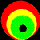Doesn’t matter if you limit the operations you can use. If you just add, you can go into decimals. 4+1=5, 4.5+.5=5, 4.00001+.00009=5. You can take those decimals out to any length and you’ve got a new expression and you’re still just adding.

theichibun (2237)“Great Answer” (1) Flag as…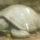@theichibun Its finite if you limit the operations and the set of possible numbers you are allowed to use. Of course, if you allow any number, you get an infinite number of equations.

@squigish‘s answer is a good way to get the number of expressions that are not “basically the same thing” in some sense.

roundsquare (5517)“Great Answer” (1) Flag as…Even if we made the reasonable restriction suggested by @squigish , although the number of ways of expressing a number is finite, there is no formula for computing it. One reason for this is that there is no formula for finding the ways of factoring a number. Suppose instead we limited ourselves to addition of positive numbers. You would think that this is something that could be computed, but it depends on what you want to consider. If you are willing to consider the order of the terms as making a difference (4+3 and 3+4 as being different) then there are computational techniques available, though there is no simple formula. If, however, you want the order of the terms as not to make a difference, so that 4+3 is the same as 3+4, then there is a hairy infinite series that converges to the answer. See Link You should be able to follow the first paragraph or two. Then do a search on “circle method” to find the equations used.

LostInParadise (28468)“Great Answer” (1) Flag as…If you restrict to only adding positive numbers, and you take into account order of numbers (e.g. 1 + 2 is different from 2 + 1) I think you can say that the number of ways to get to n is 2^(n-1).

Think about it this way. Take n balls and put them in a row. Start from the left and move right, and between each pair of balls, you can either draw or line or not. At the end there are 2^(n-1) possible ways you might have drawn the lines. Between each pair of lines is a group of balls and this gives you a unique expression for adding up to n. I.e. if the left most group has x balls, the first part of your expression is x. If the next group has y balls, you got x + y. And so on.

roundsquare (5517)“Great Answer” (3) Flag as…@roundsquare , I wish I had thought of that. GA For some reason, I thought it was more complicated. You never know what you can learn at Fluther.

LostInParadise (28468)“Great Answer” (1) Flag as…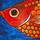Just to muddy the waters a bit I would guess, though I’m no mathematician, that infinity wouldn’t quite cover it and you would have to go to one of the higher infinities discovered by Georg Cantor if you included irrational numbers as well.

flutherother (30681)“Great Answer” (0) Flag as…@flutherother You are certainly correct. E.g. even if you ask how many ways are there to add two numbers (say x and y) and get the number 1 then your answer depends on what x and y can be:

if x and y can be only integers or rational numbers then your answer is aleph 0.
if x and y can be any rational number then your answer is aleph 1

the answers don’t change if you allow more than two terms or even a countably infinite number of terms.

im not sure it makes sense to allow an uncountably infinite number of terms. it might be possible with concepts from (advanced) calculus or analysis but i’m not sure at all

roundsquare (5517)“Great Answer” (0) Flag as…## Answer this questionor

#### Join

to answer.

This question is in the General Section. Responses must be helpful and on-topic.

Your answer will be saved while you login or join.
Have a question? Ask Fluther!
What do you know more about?
or
Knowledge Networking @ Fluther